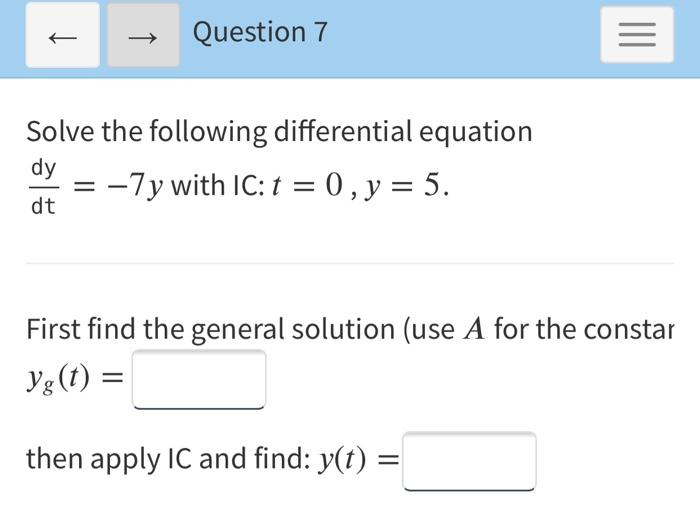### Create an Account

Already have account?

### Forgot Your Password ?

Home / Questions / → Question 7 = Solve the following differential equation dy -7y with IC:t = 0, y = 5. dt F...

# → Question 7 = Solve the following differential equation dy -7y with IC:t = 0, y = 5. dt First find the general solution (use A for the constar Yg(t) = then apply IC and find: y(t) =

→ Question 7 = Solve the following differential equation dy -7y with IC:t = 0, y = 5. dt First find the general solution (use A for the constar Yg(t) = then apply IC and find: y(t) =Apr 15 2021 View more View Less

#### Answer (Solved)Subscribe To Get Solution